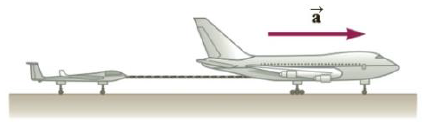Chapter 4, Problem 41P

Chapter
Section
Textbook Problem

A 276-kg glider is being pulled by a 1 950-kg jet along a horizontal runway with an acceleration of a → = 2.20 m/s2 to the right as in Figure P4.41. Find (a) the thrust provided by the jet’s engines and (b) the magnitude of the tension in the cable connecting the jet and glider.Figure P4.41

(a)

To determine
The thrust provided by the jet’s engines.

Explanation

Given Info: Mass of the jet is 1950 kg. Its acceleration is 2.20ms2 . Mass of the glider is 276 kg.

The free body diagram is given below.

In the above diagram,

• Ft is the thrust.
• N is the normal force.
• g is the acceleration due to gravity.
• a is the acceleration.
• mt is the total mass.

From Newton’s second law, formula for the thrust force is,

Ft=mta

Total mass is,

mt=1950kg+276kg=2226kg

Substitute 2226 kg for mt and 2

(b)

To determine
The magnitude of tension on the cable.

Still sussing out bartleby?

Check out a sample textbook solution.

See a sample solution

The Solution to Your Study Problems

Bartleby provides explanations to thousands of textbook problems written by our experts, many with advanced degrees!

Get Started

26-50 How do cells repair mutations caused by X-rays?

Introduction to General, Organic and Biochemistry

What would the spectrum of an auroral display look like? Why?

Horizons: Exploring the Universe (MindTap Course List)

What is the maximum number of bonds that a carbon atom can form?

Biology: The Dynamic Science (MindTap Course List)